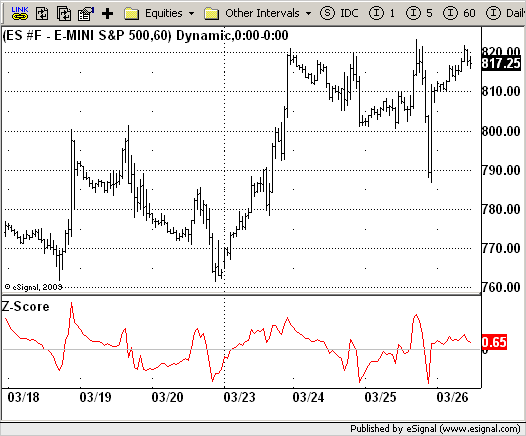# Z-Score

ICE Data Services -

Z_Score.efs
EFSLibrary - Discussion Board

File Name: Z_Score.efs

Description:
Z-Score

Formula Parameters:

• Period : 20

Notes:

The author of this indicator is Veronique Valcu. The z-score (z) for a data item x measures the distance (in standard deviations StdDev) and direction of the item from its mean (U):

`z = (x-StdDev) / U`

A value of zero indicates that the data item x is equal to the mean U, while positive or negative values show that the data item is above (x>U) or below (x Values of +2 and -2 show that the data item is two standard deviations above or below the chosen mean, respectively, and over 95.5% of all data items are contained within these two horizontal references (see Figure 1). We substitute x with the closing price C, the mean U with simple moving average (SMA) of n periods (n), and StdDev with the standard deviation of closing prices for n periods, the above formula becomes:

`Z_score = (C - SMA(n)) / StdDev(C,n)`

The z-score indicator is not new, but its use can be seen as a supplement to Bollinger bands. It offers a simple way to assess the position of the price vis-a-vis its resistance and support levels expressed by the Bollinger Bands. In addition, crossings of z-score averages may signal the start or the end of a tradable trend. Traders may take a step further and look for stronger signals by identifying common crossing points of z-score, its average, and average of average.

Z_Score.efsEFS Code:

```/*********************************
Provided By:
eSignal (Copyright c eSignal), a division of Interactive Data
Formula Script (EFS) is for educational purposes only and may be
modified and saved under a new file name.  eSignal is not responsible
for the functionality once modified.  eSignal reserves the right
to modify and overwrite this EFS file with each new release.

Description:
Z-Score

Version:            1.0  03/24/2009

Formula Parameters:                     Default:
Period                              20

Notes:
The author of this indicator is Veronique Valcu. The z-score (z) for a data
item x measures the distance (in standard deviations StdDev) and direction
of the item from its mean (U):
z = (x-StdDev) / U
A value of zero indicates that the data item x is equal to the mean U, while
positive or negative values show that the data item is above (x>U) or below
(x Values of +2 and -2 show that the data item is two standard deviations
above or below the chosen mean, respectively, and over 95.5% of all data
items are contained within these two horizontal references (see Figure 1).
We substitute x with the closing price C, the mean U with simple moving
average (SMA) of n periods (n), and StdDev with the standard deviation of
closing prices for n periods, the above formula becomes:
Z_score = (C - SMA(n)) / StdDev(C,n)
The z-score indicator is not new, but its use can be seen as a supplement to
Bollinger bands. It offers a simple way to assess the position of the price
vis-a-vis its resistance and support levels expressed by the Bollinger Bands.
In addition, crossings of z-score averages may signal the start or the end of
a tradable trend. Traders may take a step further and look for stronger signals
by identifying common crossing points of z-score, its average, and average of average.
**********************************/

var fpArray = new Array();
var bInit = false;

function preMain() {
setStudyTitle("Z-Score");
setCursorLabelName("Z-Score", 0);
setDefaultBarFgColor(Color.red, 0);

var x=0;
fpArray[x] = new FunctionParameter("Period", FunctionParameter.NUMBER);
with(fpArray[x++]){
setLowerLimit(1);
setDefault(20);
}
}

var xZ_Score = null;

function main(Period) {
var nBarState = getBarState();
var nZ_Score = 0;

if (nBarState == BARSTATE_ALLBARS) {
if(Period == null) Period = 20;
}
if (bInit == false) {
xZ_Score = efsInternal("Calc_Z_Score", Period, sma(Period), close());
bInit = true;
}

nZ_Score = xZ_Score.getValue(0);
if (nZ_Score == null) return;
return nZ_Score;
}

function Calc_Z_Score(Period, xMA, xClose) {
var nRes = 0;
var StdDev = 0;
var SumSqr = 0;
var counter = 0;
if(xMA.getValue(0) == null) return;
for(counter = 0; counter < Period; counter++)
SumSqr += Math.pow((xClose.getValue(-counter) - xMA.getValue(0)), 2);

StdDev = Math.sqrt(SumSqr / Period);

nRes = (xClose.getValue(0) -  xMA.getValue(0)) / StdDev;
return nRes;
}```